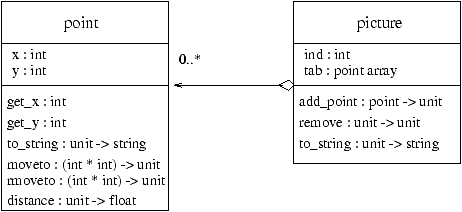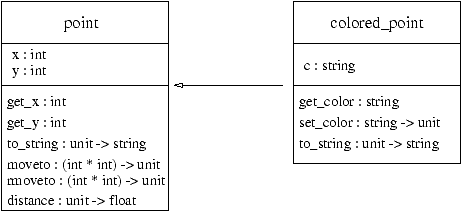## Relations between Classes

Classes can be related in two ways:
1. An aggregation relation, named Has-a:
class C2 is related by Has-a with class C1 when C2 has a field whose type is that of class C1. This relation can be generalized as: C2 has at least one field whose type is that of class C1.
2. An inheritance relation, named Is-a:
class C2 is a subclass of class C1 when C2 extends the behavior of C1. One big advantage of object-oriented programming is the ability to extend the behavior of an existing class while reusing the code written for the original class. When a class is extended, the new class inherits all the fields (data and methods) of the class being extended.

### Aggregation

Class C1 aggregates class C2 when at least one of its instance variables has type C2. One gives the arity of the aggregation relation when it is known.

#### An Example of Aggregation

Let us define a figure as a set of points. Therefore we declare class picture (see figure 15.2), in which one of the fields is an array of points. Then the class picture aggregates point, using the generalized relation Has-a.
```# class` `picture` `n` ``=`` `` `` `object` `` `` `` `` `val` `mutable` `ind` ``=`` ``0`` `` `` `` `` `val` `tab` ``=`` `Array.create` `n` `(new` `point(`0``,``0`))` `` `` `` `` `method` `add` `p` ``=`` `` `` `` `` `` `` `` `` `try` `` `tab`.`(ind)`<-`p` `;` `ind` ``<-`` `ind` ``+`` ``1`` `` `` `` `` `` `` `` `with` `Invalid_argument(`"Array.set"`)` `` `` `` `` `` `` `` `` `` `` `` `` `` `` `->` `failwith` `(`"picture.add:ind ="`` ``^`` `(string_of_int` `ind))` `` `` `` `` `method` `remove` `()` ``=`` `if` `(ind` ``>`` ``0`)` `then` `ind` ``<-`ind`-``1`` `` `` `` `` `method` `to_string` `()` ``=`` `` `` `` `` `` `` `` `let` `s` ``=`` `ref` ``"["`` `` `` `` `` `` `` `` `in` `for` `i`=``0`` `to` `ind`-``1`` `do` `s`:=`` ``!`s` ``^`` ``" "`` ``^`` `tab`.`(i)#to_string()` `done` `;` `` `` `` `` `` `` `` `` `` `(`!`s)` ``^`` ``"]"`` `` `` `end` `;;`class picture :``  int ->``  object``    val mutable ind : int``    val tab : point array``    method add : point -> unit``    method remove : unit -> unit``    method to_string : unit -> string``  end`

```

To build a figure, we create an instance of class picture, and insert the points as required.
```# let` `pic` ``=`` `new` `picture` ``8`;;`val pic : picture = <obj>`# pic#add` `p1;` `pic#add` `p2;` `pic#add` `p3;;`- : unit = ()`# pic#to_string` `();;`- : string = "[ ( 0, 0) ( 3, 4) ( 3, 0)]"`

```

#### A Graphical Notation for Aggregation

The relation between class picture and class point is represented graphically in figure 15.2. An arrow with a diamond at the tail represents aggregation. In this example, class picture has 0 or more points.Figure 15.2: Aggregation relation.

Furthermore, we show above the arrow the arity of the relation.

### Inheritance Relation

This is the main relation in object-oriented programming. When class c2 inherits from class c1, it inherits all fields from the parent class. It can also define new fields, or redefine inherited methods to specialize them. Since the parent class has not been modified, the applications using it do not need to be adapted to the changes introduced in the new class.

The syntax of inheritance is as follows:

### Syntax

inherit name1 p1 ...pn [ as name2 ]
Parameters p1, ..., pn are what is expected from the constructor of class name1. The optional keyword as associates a name with the parent class to provide access to its methods. This feature is particularly useful when the child class redefines a method of the parent class (see page ??).

#### An Example of Simple Inheritance

Using the classic example, we can extend class point by adding a color attribute to the points. We define the class colored_point inheriting from class point. The color is represented by the field c of type string. We add a method get_color that returns the value of the field. Finally, the string conversion method is overridden to recognize the new attribute.

### Note

The x and y variables seen in to_string are the fields, not the class initialization arguments.
```# class` `colored_point` `(x`,`y)` `c` ``=`` `` `` `object` `` `` `` `` `inherit` `point` `(x`,`y)` `` `` `` `` `val` `mutable` `c` ``=`` `c` `` `` `` `` `method` `get_color` ``=`` `c` `` `` `` `` `method` `set_color` `nc` ``=`` `c` ``<-`` `nc` `` `` `` `` `method` `to_string` `()` ``=`` ``"( "`` ``^`` `(string_of_int` `x)` ``^`` `` `` `` `` `` `` `` `` `` `` `` `` `` `` `` `` `` `` `` `` `` `` `` `` `` `` `` ``", "`` ``^`` `(string_of_int` `y)` ``^`` ``")"`` ``^`` `` `` `` `` `` `` `` `` `` `` `` `` `` `` `` `` `` `` `` `` `` `` `` `` `` `` ``" ["`` ``^`` `c` ``^`` ``"] "`` `` `` `end` `;;`class colored_point :``  int * int ->``  string ->``  object``    val mutable c : string``    val mutable x : int``    val mutable y : int``    method distance : unit -> float``    method get_color : string``    method get_x : int``    method get_y : int``    method moveto : int * int -> unit``    method rmoveto : int * int -> unit``    method set_color : string -> unit``    method to_string : unit -> string``  end`

```

The constructor arguments for colored_point are the pair of coordinates required for the construction of a point and the color for the colored point.

The methods inherited, newly defined or redefined correspond to the behaviors of instances of the class.
```# let` `pc` ``=`` `new` `colored_point` `(`2``,``3`)` ``"white"`;;`val pc : colored_point = <obj>`# pc#get_color;;`- : string = "white"`# pc#get_x;;`- : int = 2`# pc#to_string();;`- : string = "( 2, 3) [white] "`# pc#distance;;`- : unit -> float = <fun>`

```
We say that the class point is a parent class of class colored_point and that the latter is the child of the former.

### Warning

When redefining a method in a child class, you must respect the method type defined in the parent class.

#### A Graphical Notation for Inheritance

The inheritance relation between classes is denoted by an arrow from the child class to the parent class. The head of the arrow is a closed triangle. In the graphical representation of inheritance, we only show the new fields and methods, and redefined methods in the child class. Figure 15.3 displays the relation between class colored_point and its parent point.Figure 15.3: Inheritance Relation.

Since it contains additional methods, type colored_point differs from type point. Testing for equality between instances of these classes produces a long error message containing the whole type of each class, in order to display the differences.
```# p1` ``=`` `pc;;`Characters 6-8:``This expression has type``  colored_point =``    < distance : unit -> float; get_color : string; get_x : int; get_y : ``      int; moveto : int * int -> unit; rmoveto : int * int -> unit;``      set_color : string -> unit; to_string : unit -> string >``but is here used with type``  point =``    < distance : unit -> float; get_x : int; get_y : int;``      moveto : int * int -> unit; rmoveto : int * int -> unit;``      to_string : unit -> string >``Only the first object type has a method get_color`

```